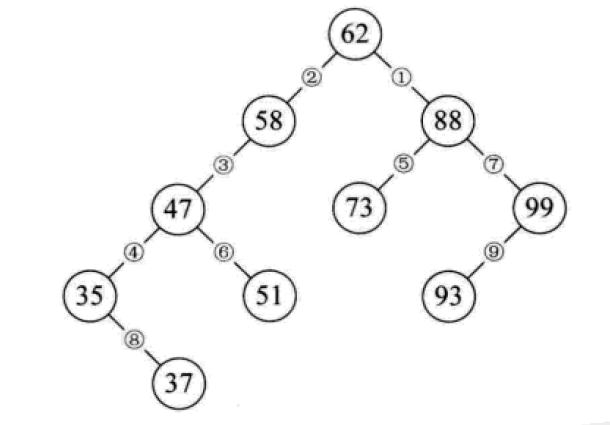# Python中的二叉排序树和平衡二叉树是什么

• python高级教程
• 发布时间：2019-06-26 17:19:05 作者：python学习 来源：py教程
• 本文有932个文字，大小约为4KB，预计阅读时间3分钟```      """
定义一个二叉树节点类。
以讨论算法为主，忽略了一些诸如对数据类型进行判断的问题。
"""
def __init__(self, data, left=None, right=None):
"""
初始化
:param data: 节点储存的数据
:param left: 节点左子树
:param right: 节点右子树
"""
self.data = data
self.left = left
self.right = rightclass BinarySortTree:
"""
基于BSTNode类的二叉排序树。维护一个根节点的指针。
"""
def __init__(self):
self._root = None
def is_empty(self):
return self._root is None
def search(self, key):
"""
关键码检索
:param key: 关键码
:return: 查询节点或None
"""
bt = self._root        while bt:
entry = bt.data            if key < entry:
bt = bt.left            elif key > entry:
bt = bt.right            else:                return entry        return None
def insert(self, key):
"""
插入操作
:param key:关键码
:return: 布尔值
"""
bt = self._root        if not bt:
self._root = BSTNode(key)            return
while True:
entry = bt.data            if key < entry:                if bt.left is None:
bt.left = BSTNode(key)                    return
bt = bt.left            elif key > entry:                if bt.right is None:
bt.right = BSTNode(key)                    return
bt = bt.right            else:
bt.data = key                return
def delete(self, key):
"""
二叉排序树最复杂的方法
:param key: 关键码
:return: 布尔值
"""
p, q = None, self._root     # 维持p为q的父节点，用于后面的链接操作
if not q:
print("空树！")            return
while q and q.data != key:
p = q            if key < q.data:
q = q.left            else:
q = q.right            if not q:               # 当树中没有关键码key时，结束退出。
return
# 上面已将找到了要删除的节点，用q引用。而p则是q的父节点或者None（q为根节点时）。
if not q.left:            if p is None:
self._root = q.right            elif q is p.left:
p.left = q.right            else:
p.right = q.right            return
# 查找节点q的左子树的最右节点，将q的右子树链接为该节点的右子树
# 该方法可能会增大树的深度，效率并不算高。可以设计其它的方法。
r = q.left        while r.right:
r = r.right
r.right = q.right        if p is None:
self._root = q.left        elif p.left is q:
p.left = q.left        else:
p.right = q.left    def __iter__(self):
"""
实现二叉树的中序遍历算法,
展示我们创建的二叉排序树.
直接使用python内置的列表作为一个栈。
:return: data
"""
stack = []
node = self._root        while node or stack:            while node:
stack.append(node)
node = node.left
node = stack.pop()            yield node.data
node = node.rightif __name__ == '__main__':
lis = [62, 58, 88, 48, 73, 99, 35, 51, 93, 29, 37, 49, 56, 36, 50]
bs_tree = BinarySortTree()    for i in range(len(lis)):
bs_tree.insert(lis[i])    # bs_tree.insert(100)
bs_tree.delete(58)    for i in bs_tree:
print(i, end=" ")    # print("\n", bs_tree.search(4))```